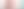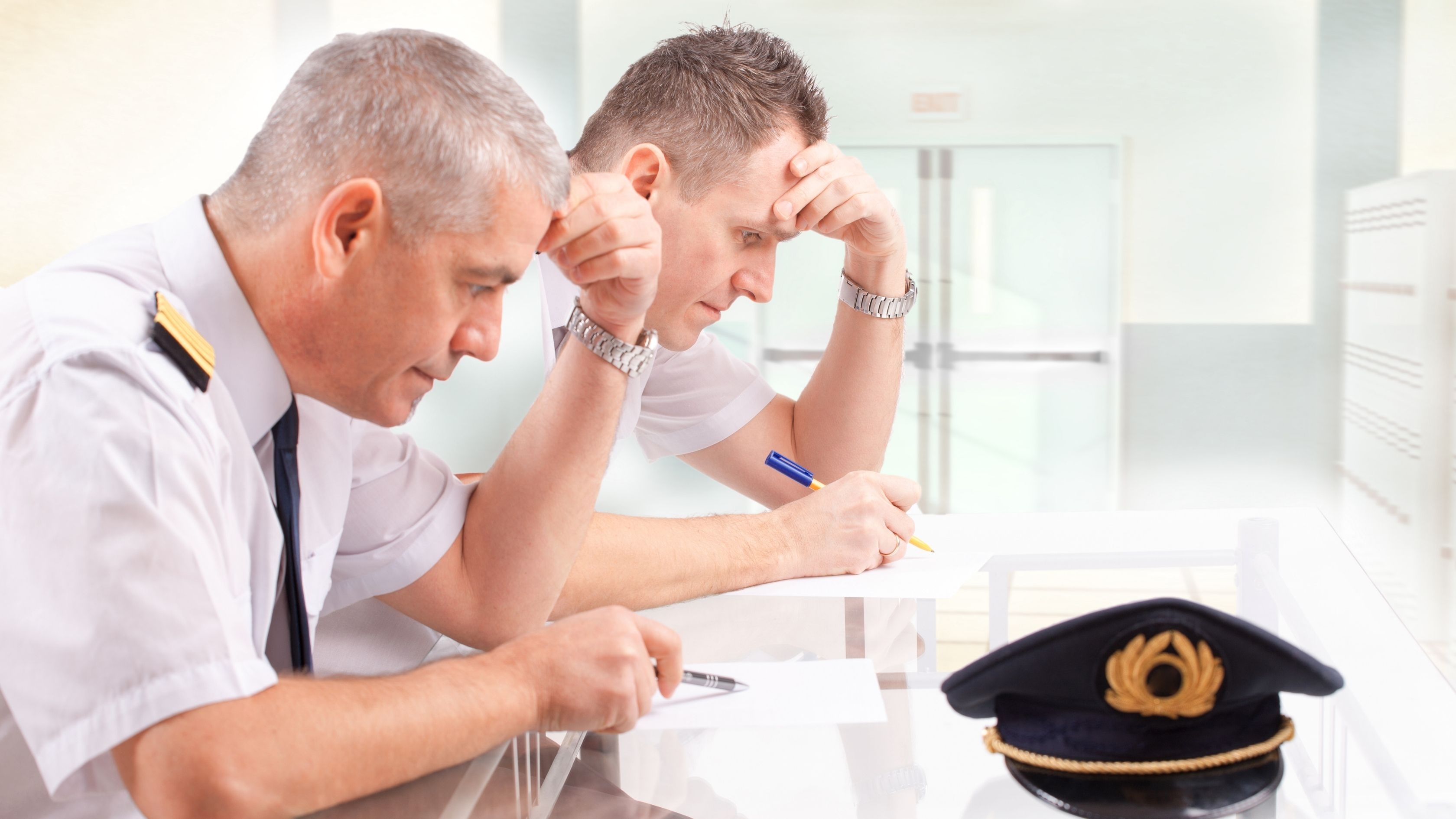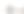Prepare for Pilots Test# Complete Guide to Speed Distance Time Questions

Updated November 15, 2023

## What Are Speed Distance Time Tests?

A form of numerical assessment, speed distance time tests measure your understanding of how the three variables of speed, distance and time relate to each other, and your ability to determine one when the other two are known to you.

They are used as part of the recruitment process for roles that require sound navigation skills and strategic thinking, such as those in the armed forces and the aviation industry.

If you are asked to sit a speed distance time test, you will need to prepare for three different question types:

• Those that require you to calculate speed, using measures of distance and time.
• Those that require you to calculate distance, using measures of time and speed.
• Those that require you to calculate time, using measures of speed and distance.

These questions are typically presented as word problems describing a scenario in which two of the variables are given.

In the sections that follow, all three question types are covered in detail, with examples for each, then guidance on how to prepare for speed distance time tests is provided.

## How Do You Calculate Speed, Distance and Time?

To calculate speed, distance and time, you need to remember three key formulas.

These are:

• Speed = Distance/Time (commonly shortened to $s = d/t$)
• Distance = Speed x Time (commonly shortened to $d = s \times t$)
• Time = Distance/Speed (commonly shortened to $t = d/s$)

For most people, the easiest way to remember these relationships is through a visual representation like the triangle formula below, where:

• 's' = speed
• 'd' = distance
• 't' = timeSpeed Distance Time Questions

Referring to the image above, you can see that covering the value you are trying to find gives you the calculation you need to perform:

• To calculate speed, cover the S, leaving D over T; so, you know you need to divide the distance by the time ($s = d/t$)
• To calculate distance, cover the D, leaving S next to T; so you know you need to multiply the speed by the time ($d = s \times t$)
• To calculate time, cover the T, leaving D over S; so you know you need to divide the distance by the speed ($t = d/s$)

### Calculating Speed

Since speed is measured using different units for distance and time (for example, miles per hour or kilometers per second) it is known as a compound measure.

To determine average speed, you need to divide the distance covered by the time taken.

In some cases, this may be a straightforward calculation.

For example, for finding the average speed of a car that traveled 120 miles in 2 hours, simply divide 120 by 2, giving an answer of 60 mph.

However, most speed distance time questions will be more complex, and you may need to convert units of time and/or distance.

Consider the example below:

Worked Example:

Calculate the average speed in miles per hour of a runner that covers 20 miles in 3 hours and 40 minutes. Give your final answer to two decimal places.

Method:

The question deals in hours and minutes, so, first, you need to find a single unit of time to work with. Converting 3 hours and 40 minutes into minutes alone gives a total of 220. This is done as follows:

$3 \times 60 = 180$
$180 + 40 = 220$

Using the formula of $s = d/t$, gives:

$20/220 = 0.0909$

Remember, you have been working in minutes, and the question asks for miles per hour. 0.0909 is the runner's miles per minute. To find their miles per hour, simply multiply that by 60.

$0.0909 \times 60 = 5.454$

The question wants the final answer to two decimal places, so round this down to 5.45.

Answer: The runner’s average speed is 5.45 mph.

When calculating speed, always make sure you are working with the correct units of both distance and time.

### Calculating Speed Sample Questions

Example Question

In his junior cup tennis match, Jack serves an Ace. The ball lands 71ft from where it was served and takes 0.5 seconds to do so.

What was the speed of Jack’s serve in mph? Give your answer to two decimal places.

Example Question

Two cars make the same journey from point A to point B. Car One takes 1 hour and 10 minutes to arrive at point B and Car Two takes 1 hour and 20 minutes.

How many mph faster did Car One travel?

If you need to prepare for a number of different employment tests and want to outsmart the competition, choose a Premium Membership from JobTestPrep.

You will get access to three PrepPacks of your choice, from a database that covers all the major test providers and employers and tailored profession packs.

### Calculating Distance

To calculate distance, multiply speed by time. This is fairly straightforward, but, again, you may need to convert units of time.

Here is an example with how to answer it broken down:

Worked Example:

If Janet walks from her house to the train station at an average speed of 5 kilometers per hour, and it takes her 20 minutes to get there, how far away from the train station does she live? Give your answer to two decimal places.

Method:

From the information given here, you know how fast Janet walks per hour, but to answer the question, you need to know her walking speed per minute.

To calculate this, you can simply divide 5 by 60, as there are 60 minutes in an hour:

$5/60 = 0.083$

Now you know Janet walks at an average speed of 0.083 kilometers per minutes, you can use the formula of $d = s \times t$:

$0.083 \times 20 = 1.66$

Answer: Janet lives 1.66 km away from the train station.

### Calculating Distance Sample Questions

Example Question

Sarah takes part in the javelin on sports day. In her best throw, the javelin travels at an average speed of 12 meters per minute and is in the air for 6 seconds. What was the distance of Sarah’s best throw in meters? Give your answer to two decimal places.

Example Question

Tim completes a charity bike ride over the course of two days. On day one, he cycles at an average speed of 10 mph for 5 hours. On day two, he cycles for 6 hours at an average speed of 12 mph.

How far was the bike ride in total?

### Calculating Time

To calculate time, you divide the distance by speed. Again, pay close attention to any other calculations you need to perform to answer the question correctly.

This could be converting units of distance, such as changing mph to km/h, or converting time, as in the example below:

Worked Example:

A high-speed train travels directly from point A to point B at an average speed of 200 mph. The distance between point A and point B is 545 miles. How long does it take the train to cover the distance? Give your answer in hours, minutes and seconds.

Method:

First, apply the formula: $t = d/s$:

$545/200 = 2.725$

2.725 is in hours only because the average speed was given as miles per hour.

Convert 2.725 into hours, minutes and seconds by multiply the partial hours of .725 by 60 to get their equivalent in minutes:

$0.725 \times 60 = 43.5$

This still does not give a whole number, so the seconds must be calculated too.

Multiply the fractional minutes of .5 by 60 to get their equivalent in seconds:

$0.5 \times 60 = 30$

Answer: The train took 2 hours, 43 minutes and 30 seconds to cover the distance.

### Calculating Time Example Questions

Example Question

A commercial flight carries passengers the 3,470 miles from London to New York. If the plane travels at an average speed of 560 mph, how long will the flight take? Give your answer in minutes to the nearest whole minute.

Example Question

Gemma runs a 10 km race at an average speed of 11.2 km/h. How long did she take to complete the race? Give your answer in hours, minutes and seconds.

## How to Prepare for Speed Distance Time Tests

A strong performance on a speed distance time test all comes down to good preparation.

Here are step-by-step tips for success:

### Step 1. Practice Mental Arithmetic, Including Your Times Tables

This will help you identify number relationships at a glance, making calculations much easier and quicker to perform. The 6 times table, in particular, is very useful, since it relates to time (60 minutes in an hour, 60 seconds in a minute).

### Step 2. Memorize the Key Formulas

In speed distance and time questions, two of the variables will always be made known to you, so all you need to do is remember the correct formula to apply to find the third.

Before you begin your test, draw out the triangle formula so you have a clear point of reference throughout.

### Step 3. Get Comfortable With Conversions

As in some of our example questions, speed distance time tests often require you to convert units.

In some cases, you may be given a measurement of speed in one unit and asked to provided distance in another, or vice versa, so you will need to be confident with conversions.

You should also brush up on converting time.

### Step 4. Focus On Working Accurately, but Quickly

It is likely that your test will be timed, and that you will be assessed on both the accuracy of your answers and the speed with which you completed them. Practicing is key here.

The more you work through sample questions, the quicker you will be able to identify and perform the necessary calculations.

### Step 5. Pay Close Attention to Detail

Read every question thoroughly until you are sure of what is being asked of you. Points can be easily dropped by missing a key part of the question, such as responding in hours when you have been asked to give your answer in minutes.

Double-check the units of distance too. You may have done the math right, but if your answer states km/h when it should read mph, it is incorrect.

### Step 6. Avoid Human Error

Whether you are performing calculations with pen and paper or using a calculator, always refer back to the figures stated in the official test question.

If you have scribbled down notes, you may have missed a digit or got numbers muddled. These mistakes are easily made under pressure, so make sure you are working with figures that exactly match those in the question.

### Step 7. Use Your Own Calculator

If calculators are permitted in your speed distance time test, make sure you use one that you are familiar with.

Remember, you will likely be working under time pressure, and familiarity with your equipment can save you precious seconds.

Of course, the best tip on how to prepare for speed distance time tests is to practice. There are plenty of resources available online with sample questions and answers to work through.

For slightly broader guidance, this article discusses fractions in-depth, including how to convert them to and from percentage, which is a useful skill for unit conversion.

Or there are free numerical computation questions here to practice addition, multiplication, division and subtraction.

Further, this ebook contains 440 practice questions, albeit not exclusively about speed distance time.

The more you make use of these and practice math in general, the more competent you will become.